# 常见的五种神经网络(4)-深度信念网络（上）篇之玻尔兹曼机和受限玻尔兹曼机

• 背景
• 玻尔兹曼机
• 受限玻尔兹曼机

### 玻尔兹曼机

#### 介绍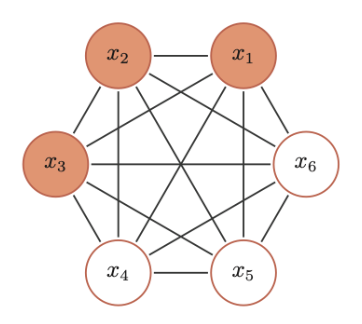BM的三个性质：

• 二值输出：每个随机变量可以用一个二值的随机变量表示
• 全连接：所有节点之间是全连接的
• 权重对称：每两个变量之间的相互影响是对称的

BM中的每个变量$X$的联合概率由玻尔兹曼分布得到，即：
$p(x) = \frac{1}{Z} exp(\frac{-E(x)}{T})$

$E(x) \overset{\bigtriangleup }{=} E(X=x) = -( \sum_{i

#### 生成模型

1. 全条件概率

$p(x_i=1|x_{\setminus i}) = \sigma( \frac{ \sum_{j} w_{ij}x_j +b_i }{T} ) \\ p(x_i=0|x_{\setminus i}) = 1- p(x_i=1|x_{\setminus i})$

2. 吉布斯采样

$x_i = \left\{\begin{matrix} 1 & if \sum_{j}w_{ij}x_j + b_i \geq 0\\ 0 & otherwise \end{matrix}\right.$

3. 能量最小化与模拟退火

### 受限玻尔兹曼机

#### 介绍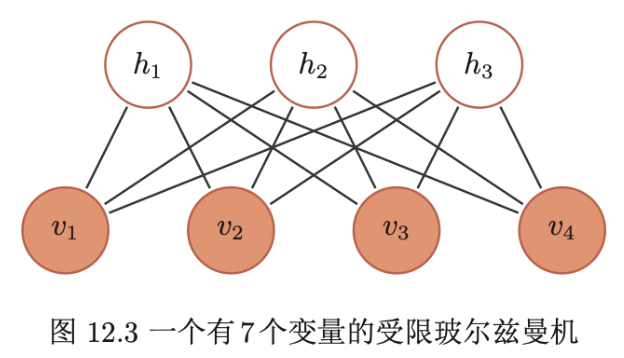• 可观测的随机向量$v=[v_1, ..., v_{m_1}]^T$
• 隐藏的随机向量 $h=[h_1, ... , h_{m_2}]^T$
• 权重矩阵$W \in R^{m_1 * m_2}$，其中每个元素$w_{ij}$为可观测变量$v_i$和隐变量$h_j$之间边的权重
• 偏置$a \in R^{m_1}$$b \in R^{m_2}$，其中$a_i$为每个可观测变量$v_i$得偏置，$b_j$为每个隐变量$h_j$得偏置

$E(v,h) = - \sum_{i} a_iv_i - \sum_{j}b_j h_j - \sum_{i}\sum_{j}v_i w_{ij}h_j = -a^Tv -b^Th - v^T W h$

$p(v,h) = \frac{1}{Z} exp(-E(v,h)) = \frac{1}{Z} exp(a^Tv)exp(b^Th)exp(v^TWh)$

#### 生成模型

1. 全条件概率

$p(v_i | v_{\setminus i},h) = p(v_i|h) \\ p(h_j | v,h_{\setminus j}) = p(h_j|v)$

$p(v_i=1|h) = \sigma (a_i + \sum_{j}w_{ij} h_j) \\ p(h_j=1|v) = \sigma (b_j + \sum_{i}w_{ij} v_i)$

2. RBM中得吉布斯采样

• （给定）或随机初始化一个可观测的向量$v_0$，计算隐变量得概率，并从中采样一个隐向量$h_0$
• 基于$h_0$，计算可观测变量得概率，并从中采样一个个可观测的向量$v_1$
• 重复$t$次后，获得$(v_t, h_t)$
• $t \rightarrow \infty$时，$(v_t,h_t)$的采样服从$p(v,h)$分布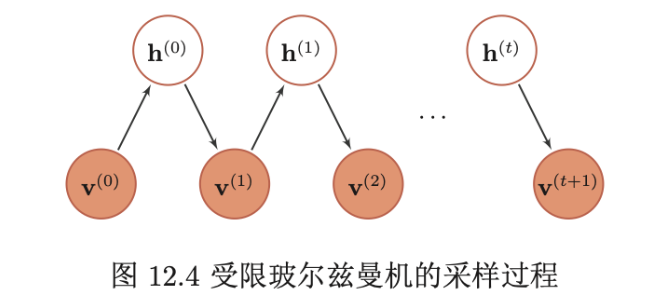3. 对比散度学习算法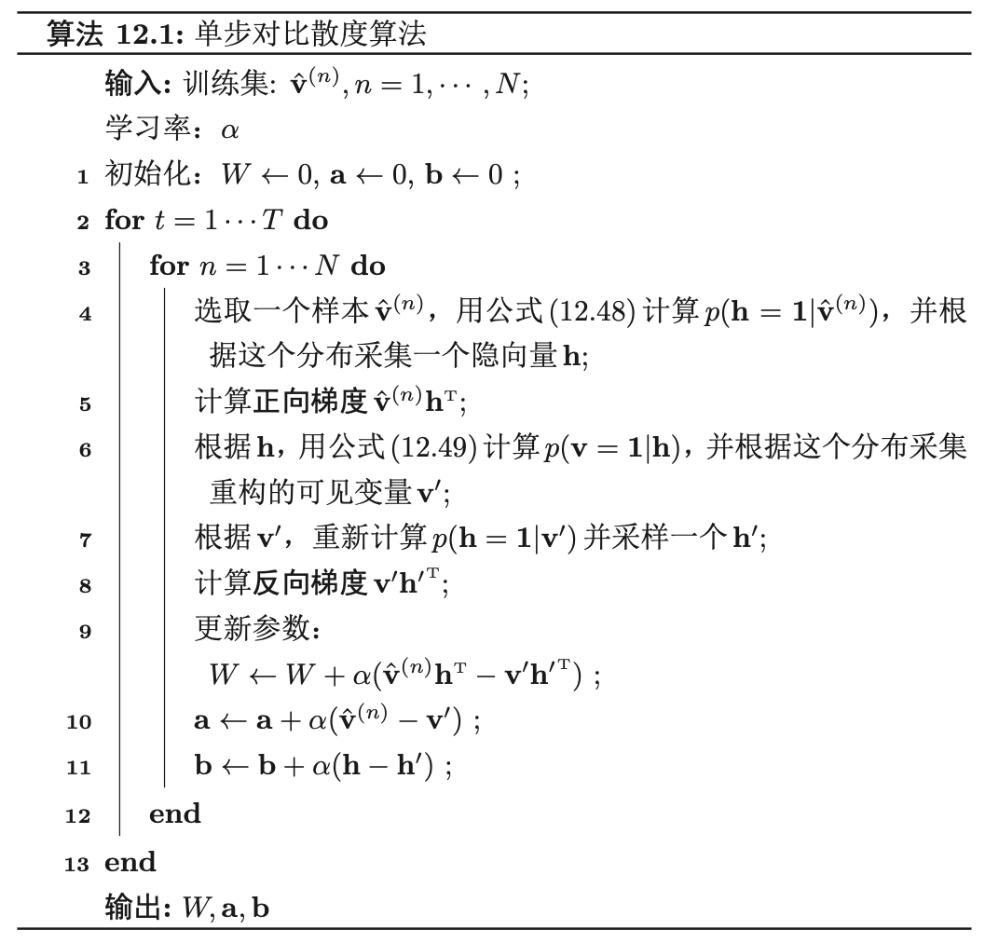4. 受限玻尔兹曼机得类型

• “伯努利-伯努利”受限玻尔兹曼机：即上面介绍得可观测变量喝隐变量都为二值类型得受限玻尔兹曼机
• “高斯-伯努利”受限玻尔兹曼机：假设可观测变量为高斯分布，隐变量为伯努利分布，其能量函数定义为：
$E(v,h) = \sum_{i} \frac{(v_i - \mu_i)^2}{2 \sigma_i^2} - \sum{j} b_jh_j - \sum_{i}\sum{j} \frac{v_i}{\sigma_i}w_ijh_j$
其中每个可观测变量$v_i$服从$(\mu_i, \sigma_i)$的高斯分布。
• “伯努利-高斯”受限玻尔兹曼机：假设可观测变量为伯努利分布，隐变量为高斯分布，其能量函数定义为：
$E(v,h)=\sum_{i}a_i v_j - \sum_{j} \frac{(h_j-u_j)^2}{2\sigma_j^2} - \sum_{i}\sum_{j}v_iw_{ij}\frac{h_j}{\sigma_j}$
其中每个隐变量$h_j$服从$(\mu_j, \sigma_j)$的高斯分布【技术服务】，详情点击查看：https://mp.weixin.qq.com/s/PtX9ukKRBmazAWARprGIAg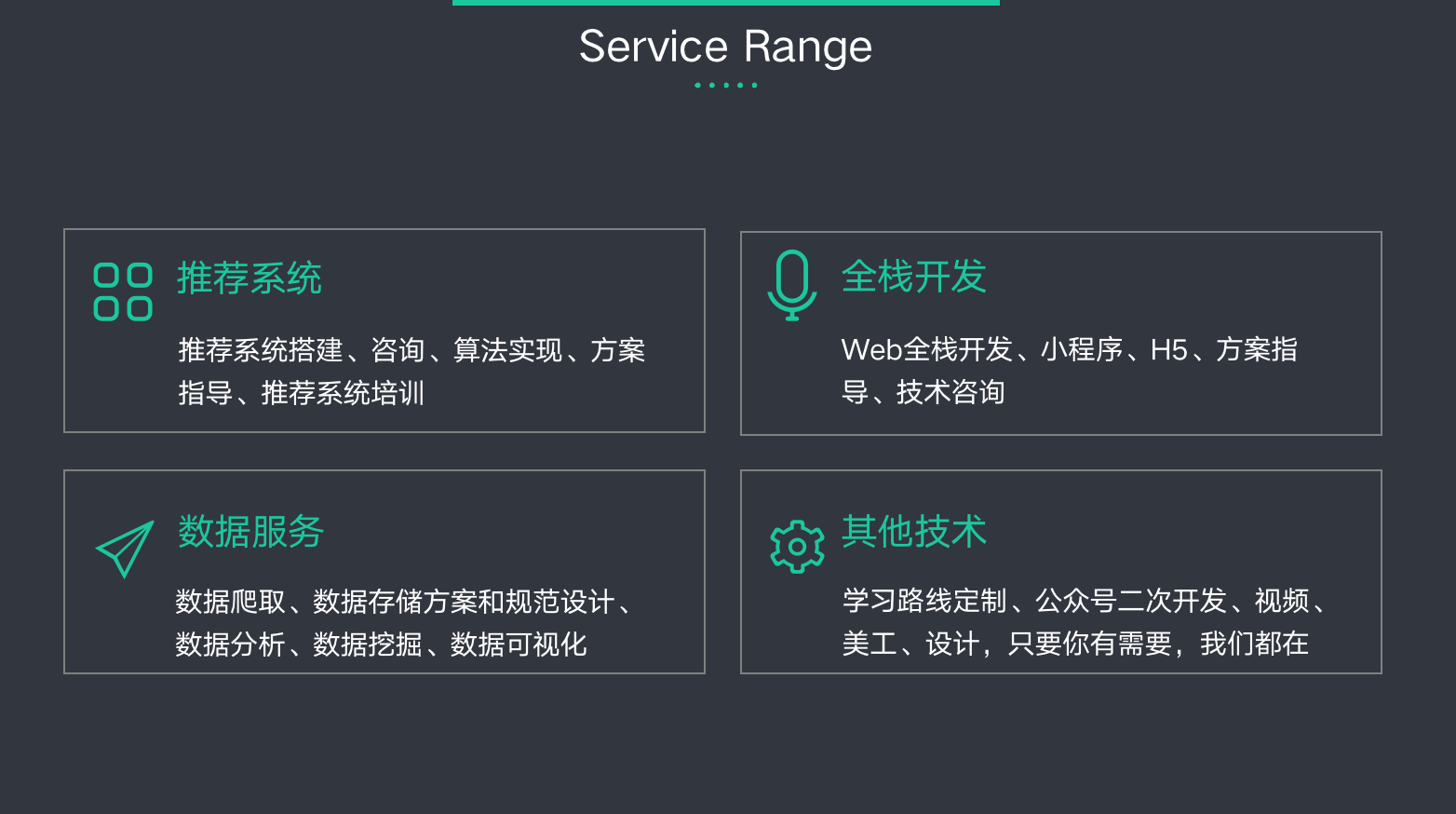©️2019 CSDN 皮肤主题: 大白 设计师: CSDN官方博客